Categories

## An Experimental Lesson

Since visiting The De Ferrers Academy a few years ago I have thought about trying to create a video using ExplainEverything. This half term was my school’s “Take a Risk” observation window. We have one of these every year and I think it’s quite a good idea as the outcome of the observation doesn’t count formally so it does encourage taking a bit more of a risk with the lesson.

For mine this time I was being observed with my Year 13 further mathematicians and we were going to be starting to look at the FP3 conic sections stuff.

My plan was to produce a video introducing the Ellipse using ExplainEverything and then provide some questions for the students to work through without any help from me.

Here is the video:

As you can see the video bears a few hallmarks from it being created at 2am and there are some things that I don’t think I explained terribly well in a mathematical sense. However, it seemed to serve its purpose well and my students were able to tackle the questions that I had given them, such as this one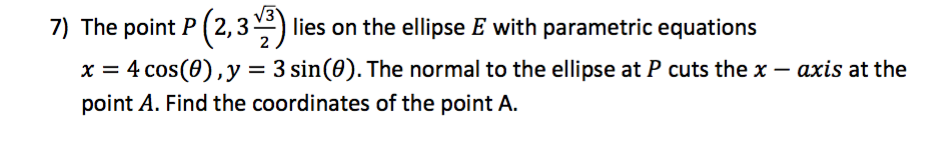I had two versions of the questions; one with more intermediate steps to guide students through the question. Thanks to Stuart (@sxpmaths) for the picture of the ellipse I used in the questions, it saved me from drawing my own.

Before the lesson my students also had access to the two Geogebra applets featured in the video, that I created and hosted on my website. The first allowed students to explore the parametrisation of the ellipse and investigate the foci property of an ellipse.The second demonstrated one method of constructing an ellipse (without equations) known as the Trammel Construction.I’ve put all the worksheets on my website and they are available as follows:

Feel free to use them if you wish.

PS:- In case you are interested, I got very good feedback for this lesson 🙂

Categories

## An Undiscovered MATLAB Function

Regular readers here will know that I love using MATLAB for some things but earlier this week I discovered a function that I hadn’t used before and is surprisingly useful.

I wanted to produce some diagrams showing portions of a normal distribution shaded and had tried to do it in Geogebra but wasn’t quite happy with the results. I then decided to try R but for some reason (probably to do with X-Windows or Quartz) the graphics output on the R installation on my Mac wasn’t working properly so I fired up MATLAB. My original intention was to plot the normal distribution function I was interested in, then plot the vertical lines at the limits and then shade the region. However, I discovered that in the “Statistics and Machine Learning Toolbox” there was a function called $$\texttt{normspec}$$.

The syntax for this function is described in detail in the documentation but essentially this function can be used to shade a region under a given normal distribution. The syntax is $$\texttt{p = normspec(specs,mu,sigma)}$$ and this plots the normal distribution with mean $$\texttt{mu}$$ and standard deviation $$\texttt{sigma}$$. It then shades the portion inside the specification limits given by the two element vector $$\texttt{specs}$$ – you can use $$\texttt{-Inf}$$ or $$\texttt{Inf}$$ if there is no lower or upper limit respectively.

For example, the command $$\texttt{p = normspec([-Inf,65],65,5)}$$ produces the plot shown below: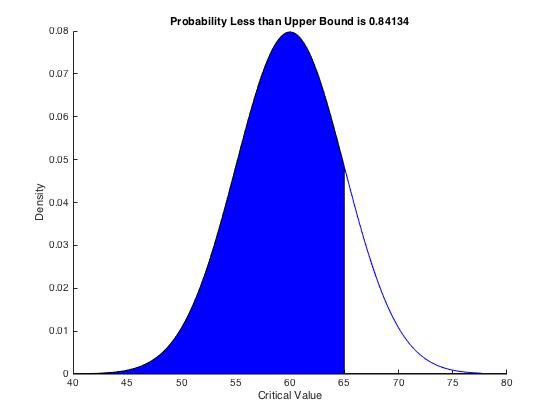I’d encourage you to go and try it out if you have a MATLAB licence.

Categories

## Barnsley’s Fern

Barnsley’s fern is a mathematically  generated image that resembles the Black Spleenwort fern as shown below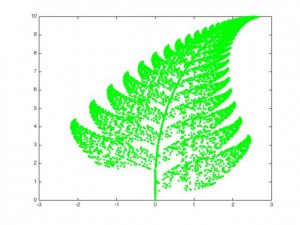This fractal was first described by Michael Barnsley in his book Fractals Everywhere in 1993. It is surprisingly easy to generate and is an example of an iterated function system. It is based on randomly applying one of four affine transformations.

\begin{align} f_1(x,y) &= \begin{pmatrix} 0.00 & 0.00 \\ 0.00 & 0.16 \end{pmatrix} \begin{pmatrix} x \\ y \end{pmatrix} + \begin{pmatrix} 0.00 \\ 0.00 \end{pmatrix} \\ f_2(x,y) &= \begin{pmatrix} 0.85 & 0.04 \\ -0.04 & 0.85 \end{pmatrix} \begin{pmatrix} x \\ y \end{pmatrix} + \begin{pmatrix} 0.00 \\ 1.60 \end{pmatrix} \\ f_3(x,y) &= \begin{pmatrix} 0.20 & -0.26 \\ 0.23 & 0.22 \end{pmatrix} \begin{pmatrix} x \\ y \end{pmatrix} + \begin{pmatrix} 0.00 \\ 1.60 \end{pmatrix} \\ f_4(x,y) &= \begin{pmatrix} -0.15 & 0.28 \\ 0.26 & 0.24 \end{pmatrix} \begin{pmatrix} x \\ y \end{pmatrix} + \begin{pmatrix} 0.00 \\ 0.44 \end{pmatrix} \end{align}

The initial point is set to be the origin, and subsequent points are generated by applying one of the above four transformations. Which transformation is chosen probabalistically – the probabilities are $$0.01,0.85,0.07$$ and $$0.07$$ for $$f_1,f_2,f_3$$ and $$f_4$$ respectively.

I wrote two (basic) functions in Matlab, one to generate the points and then one to plot them.

A video (generated using Matlab) showing the growth of the fern is below:

Here is the Matlab code I used to generate the video – it takes a relatively long time to run as it plots each frame separately.
Categories

## Geogebra and Further Maths

This week I had an observed lesson, where the remit was that I do something risky that I perhaps wouldn’t have done otherwise.

My Year 12 Further Maths class had been selected as my observation lesson – I found thinknig of something risky to do with them harder than if it had been any other group. They are used to me doing odd things, that are a bit off the wall with them and they always respond well. In the end I decided to use ICT and try to get them to do some independent discovery work.

We have just started the coordinate geometry section of FP1 (Edexcel) and wer due to look at the parabola this week. I have used GeoGebra a bit in the past but I had never created my own geogebra worksheet before and this seemed like the perfect opportunity.

So, one evening I installed the latest version of GeoGebra on my MacBook, signed up for a GeoGebra Tube account and set about creating a worksheet where students would be able to explore the parametric equations of a parabola and the focus directrix property for themselves. I then uploaded the file to GeoGebra Tube, and got the content ID from the embed option. As I didn’t want to rely on GeoGebra being installed / working on all the computers in my teaching room I decided to upload a html version to my own webpage. The GeoGebra tean have made this really easy by poviding a javascript library that you can just source at the top of your html code and then a really simple API to embed a dynamic worksheet in your webpage. They have provided examples of how to do this.The web apps for the Parabola and Hyperbola that I created are here. On loading the page you should see a screen that looks like this:I then wrote a sheet with some questions to guide the students’ explorations here, these questions should prompt them to derive the parametric equations of the parabola and notice the focus-directrix property. It is significantly harder to answer the questions concerning the hyperbola – I saw these as hard extension questions.

All of my class seemed to enjoy using these and engaged well with the work. Walking round the room I also saw some great responses to questions.

To produce the worksheets, upload to GeoGebra Tube and then host on my website took in total about 2 hours which I don’t think is bad for a first time.

My intention is to use GeoGebra moe across the keystages, any worksheets I create I will share through my website as well as GeoGebra Tube. The original GeoGebra files (in case you want to modify them) are here (parabola) and here (hyperbola). I will also be adding a worksheet for the Ellipse to the webpage later too.

Some examples of students responses to the questions are shown below:

﻿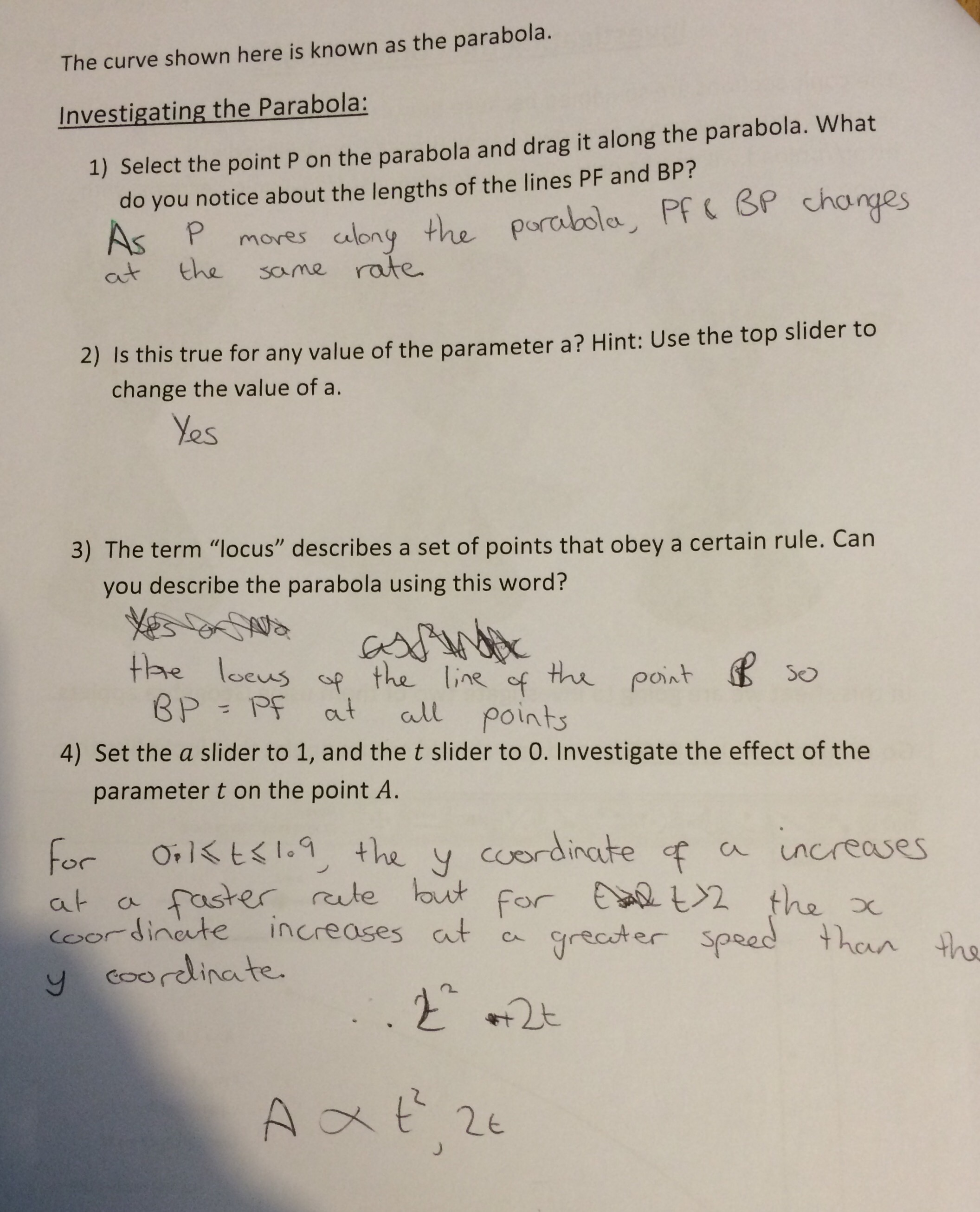﻿﻿﻿

Update: Please be aware that for the web applets to load there has to be communication with the GeoGebra website, so make sure that is not on your schools block list.

Categories

## Matlab in the Classroom

One of the things I have missed since teaching in a school is the fact that I don’t have access to Matlab….

Matlab is a product from The Mathworks, designed originally for numerical computation (indeed the name derives from MATrix LABoratory as the software was originally focussed towards operations on matrices) but which now is capable of much more. More information about Matlab and The Mathworks is available here.

I had got used to using Matlab in teaching an demonstrating, not least because of the ease with which you can use it to check matrix computations, plot graphs of functions and demonstrate numerical methods.

Last term I set my Year 12 Further Mathematicians some homework which involved them researching the Jacobi Method and performing 2 iterations of this method on a $$2 \times 2$$ matrix system. I wanted to be able to demonstrate this straightforward numerical method to them, without having to work out how to use the Python IDE on the Windows computers at school, so I decided to give Matlab Mobile a try.

Matlab Mobile was originally released in 2010 (see here for an early blog post discussing Matlab Mobile) but I hadn’t had much reason to use it before. Originally the app needed to connect to your computer with a running copy of Matlab, but since it’s release it has become a lot better. Th app is currently on version 4.1.1 which is a universal app suitable for both the iPad and the iPhone. With this version (as long as you have a valid Matlab Licence for desktop) you can connect to a copy of Matlab running in the cloud to execute commands. You can also upload your files to the cloud so that they can be run from the app.

On opening the app you are presented with a screen that looks like this: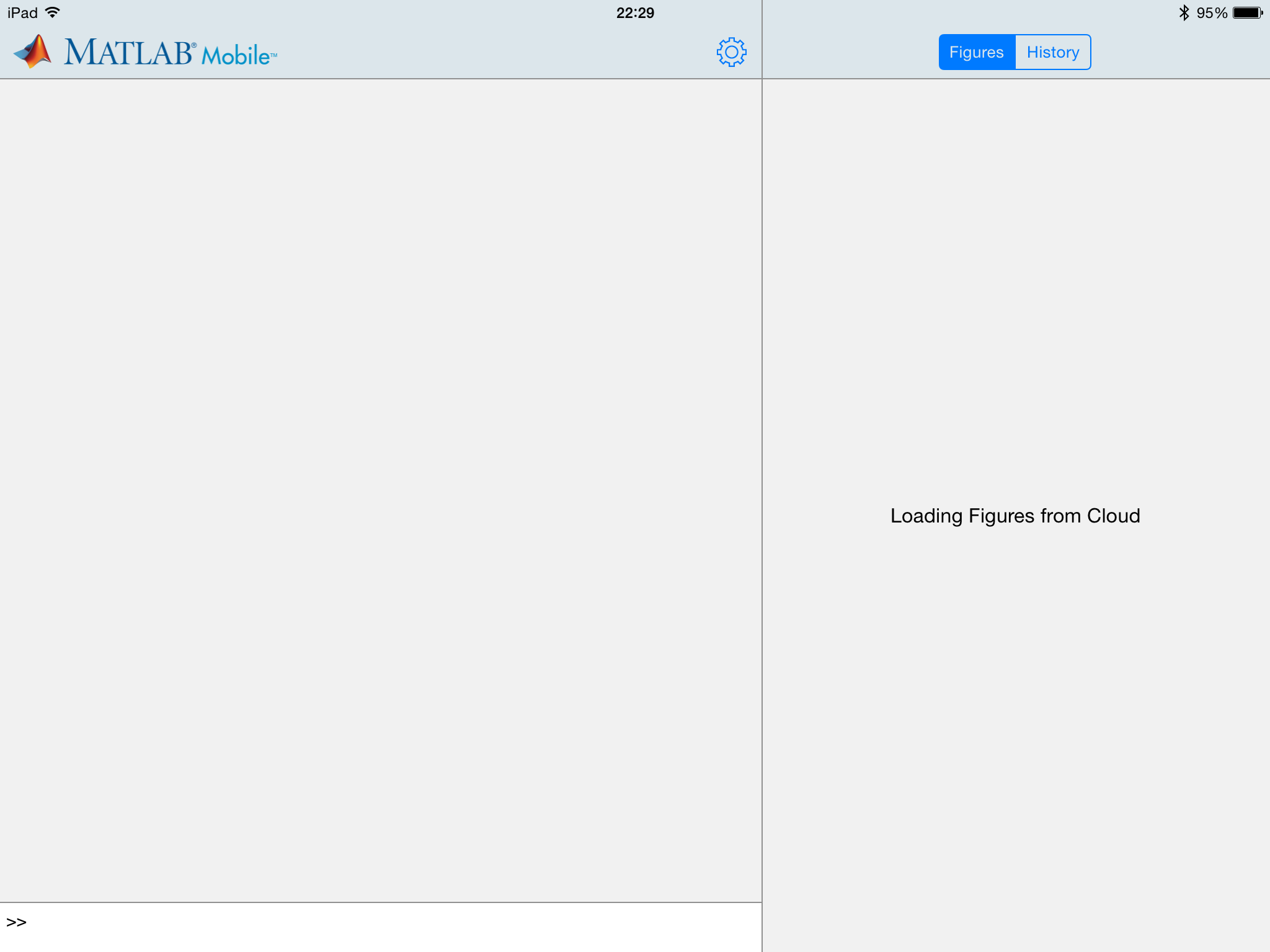The left hand portion of the screen displays commands that you have typed and on the right hand side you can toggle between Figures and viewing your command history.

Using a keyboard (I recommend using a bluetooth keyboard here as the on screen keyboard takes up so much space) it is easy to type short commands that accomplish complex things. For example, in the screen shot below, I create a matrix and then find its eigenvalues using the  eigs  command. As you can see this command can also return the corresponding eigenvectors of a matrix simply by explicitly giving two output arguments to the function.One of the great uses of the Matlab desktop product is to prototype functions before coding in another language. Unfortunately, the mobile app does not allow you to edit or create  .m  script files or function files. This is a real shame, especially as it extends to the inability to make small edits to files you have already stored in the cloud. Luckily though, you can at least view the test of functions that you have written by using the command  type  in the command window, as shown below.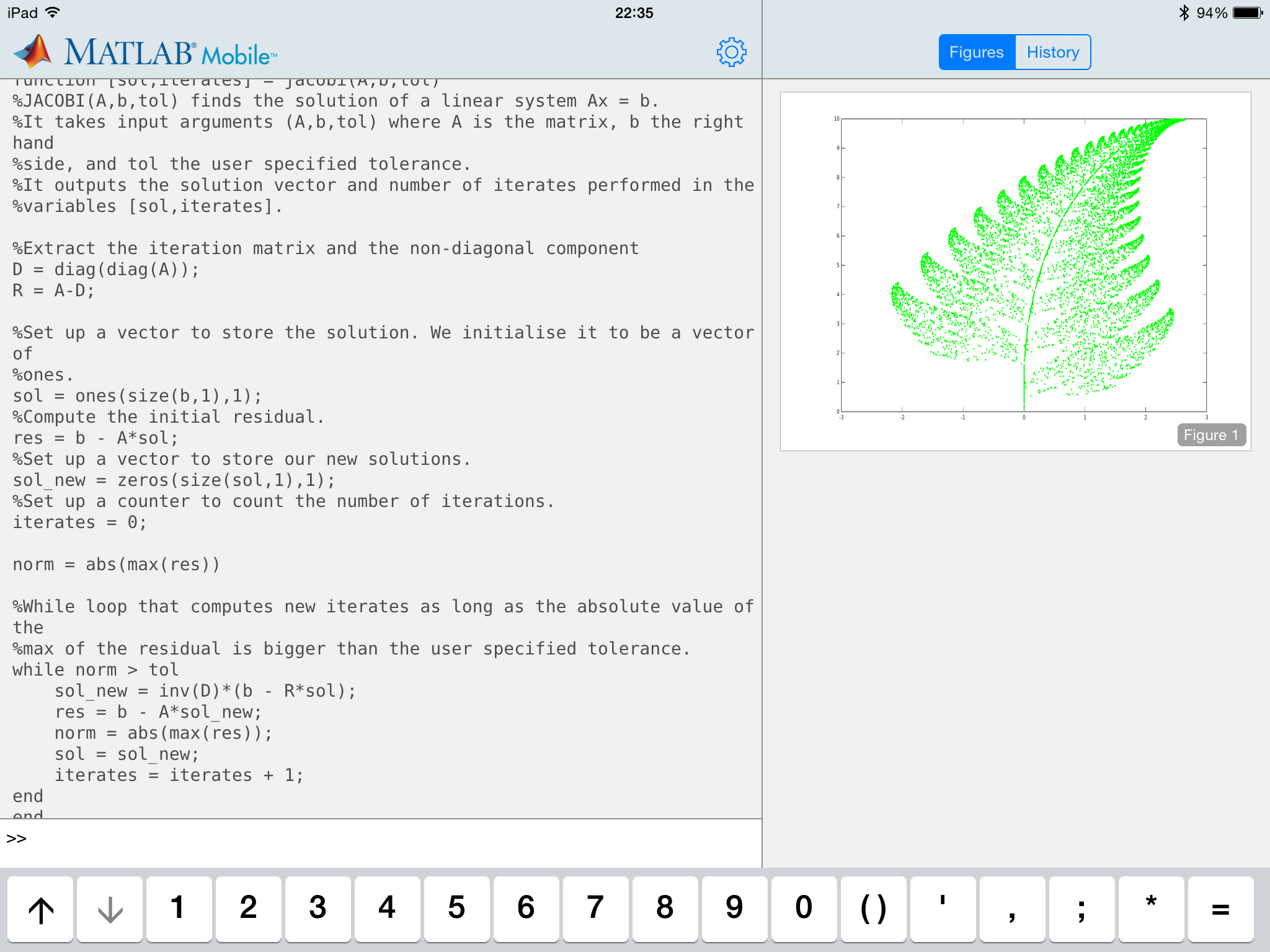The code shown above is a naive implementation of the iterative Jacobi scheme for solving systems of linear equations – I stress it is not the most efficient way to code this algorithm, but it is clear for teaching purposes. Using Matlab Mobile I was able to discuss this short function with my class, and relate it to the algorithm that they had to research and then express in pseudocode.
If you are going to use Matlab Mobile for a similar purpose I would recommend avoiding writing script files and instead use function files with enough arguments to allow you to vary everything you will want to demonstrate the affect of. I would also say it is probably useful to print more of the internal workings of the function to the command line than you would normally.

Another great piece of functionality in Matlab is the ability to produce professional looking graphs with very little effort. You can still generate figures such as the one below (a visualisation of the Barnsley fern after 10000 iterations – I may write a blog post about this in the future) in the Cloud, but a lot of the data exploration tools in the figure window of the desktop product are not usable. You can however, save the figure to your camera roll or email it to yourself.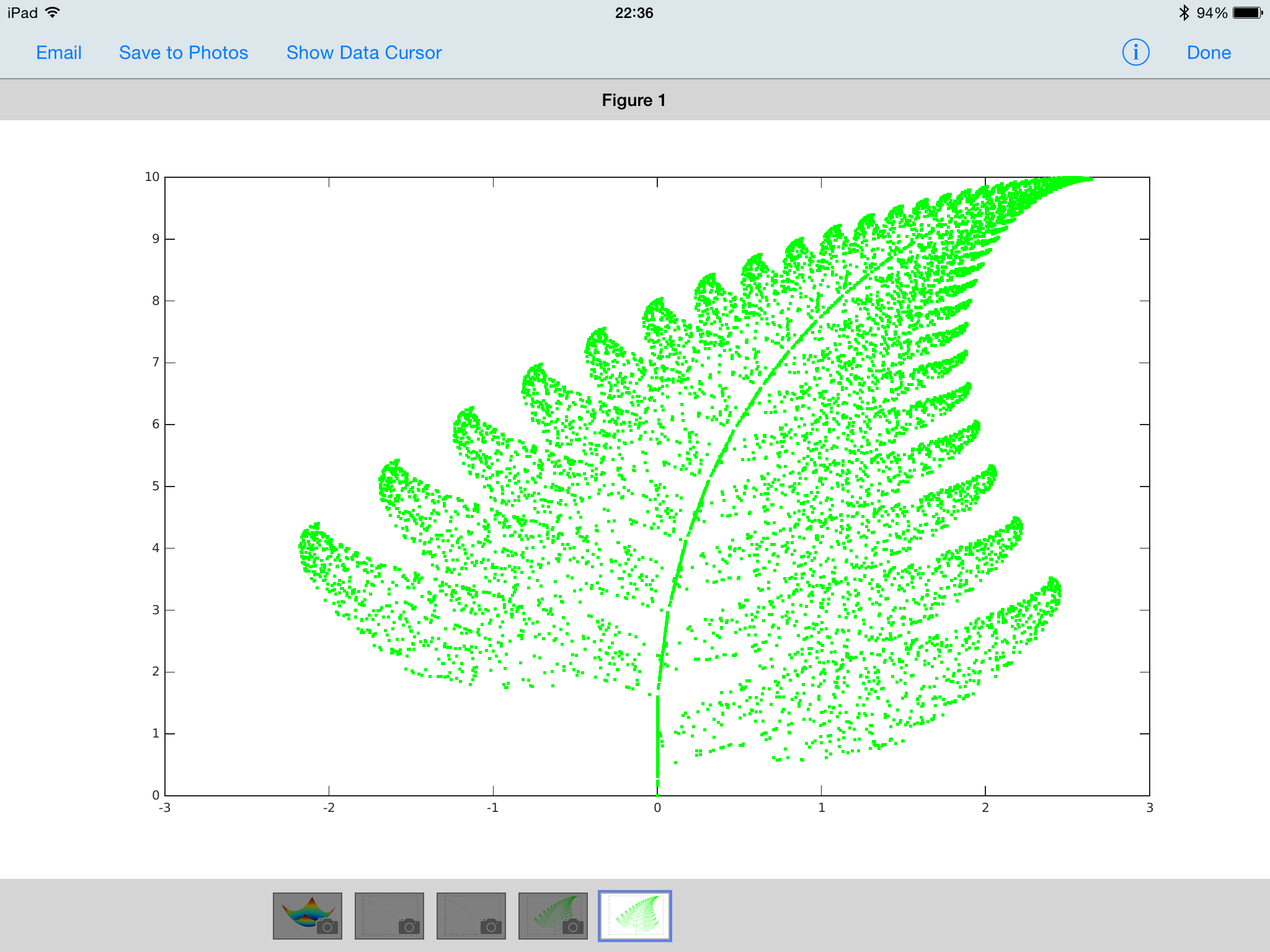As a final example of the graphical abilities of Matlab Mobile the 3D surface plot below was created using 4 lines of code.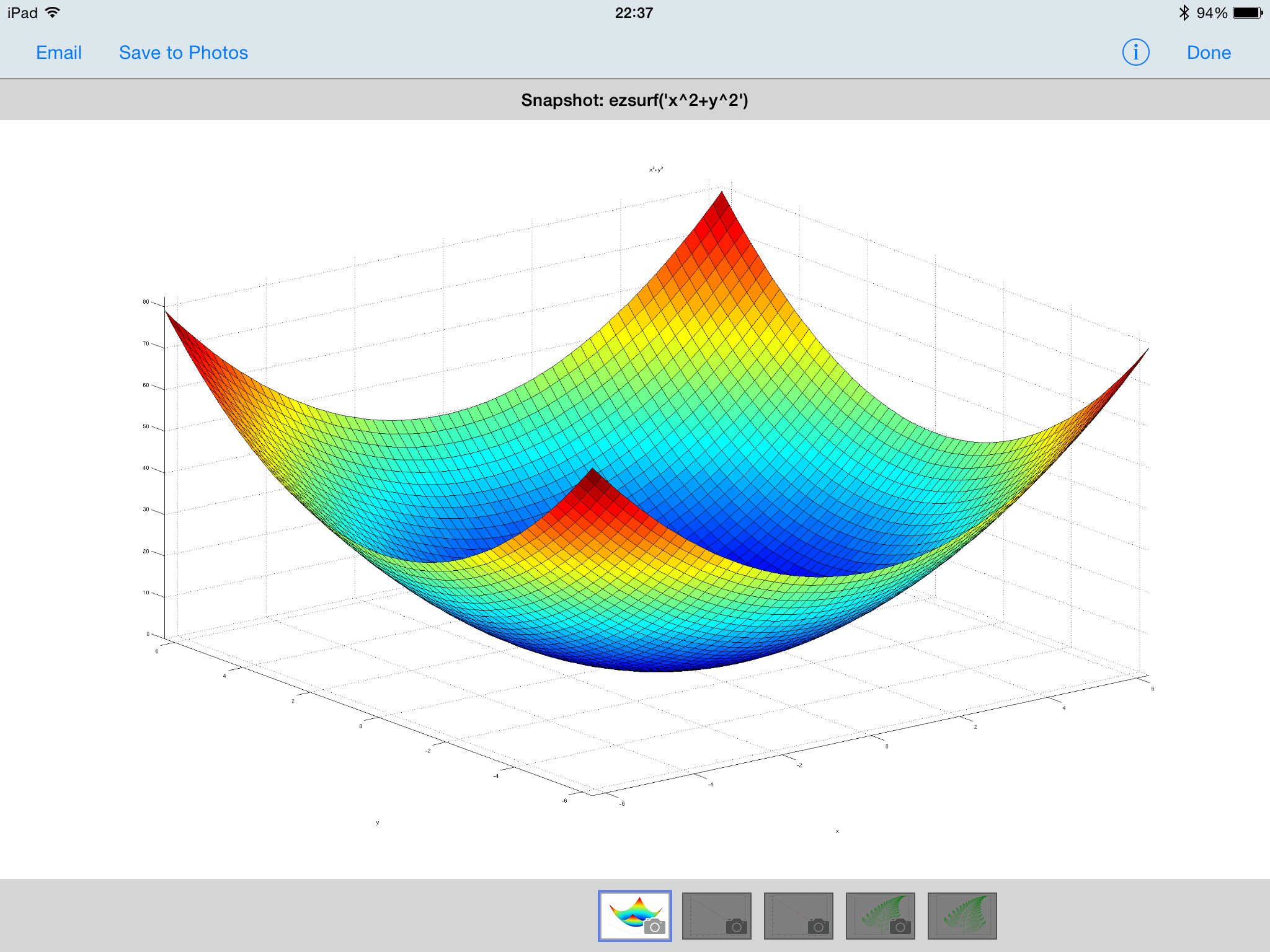One important thing to note is that I couldn’t connect to the Matlab Cloud through my schools wifi (I suspect because of the firewall) and so had to resort to using my phone as a 4G hotspot.

All in all, if you have a Matlab licence it is well worth familiarising yourself with the Mobile app, and I managed to do all that I wanted to do in the classroom with it. I just needed to do a bit more forward planning than I would have done if I had had access to the fully fledged Matlab product! Hopefully as the app develops they will remove some of the limitations that are currently present.

Categories

## Getting to know Excel

Microsoft Excel seems to be a mainstay in schools for analysing and querying all kinds of data. Until last year I had barely used Excel since I, myself, was a pupil at school. Having spent years using Matlab to display, manipulate and interrogate data of many different forms.
Getting used to using Excel I’ve found many things that irritate me (not only the fact that it is often used for serious statistics when it shouldn’t be) and I find counterintuitive.

One of these is the behaviour of the LOOKUP function. In the simple example shown below I wish to examine a table of test results sorted alphabetically to find out who scored 56. A quick google suggests LOOKUP as a common way to do this, however as you see this doesn’t work.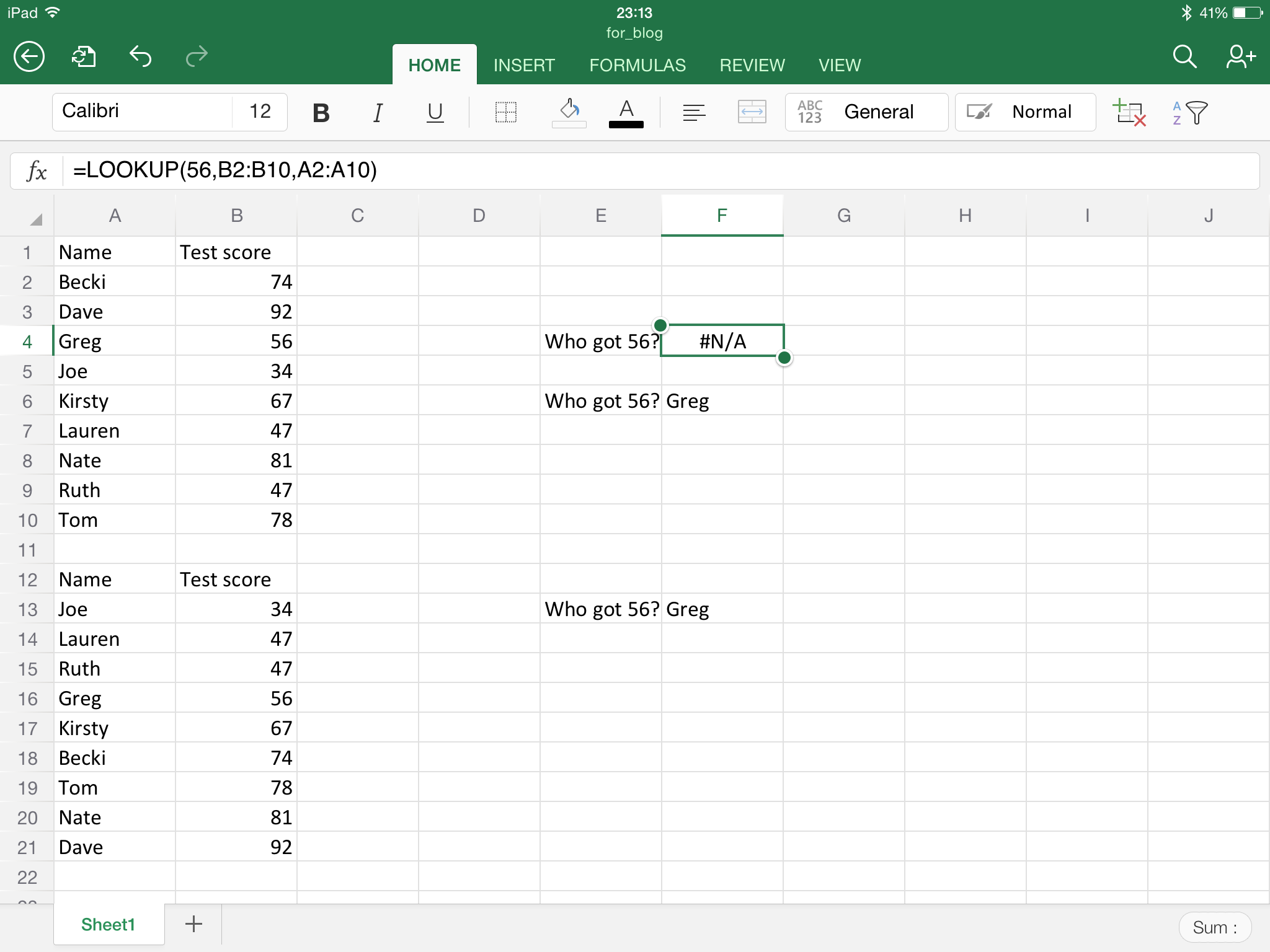It doesn’t work because the vector in which we are searching for 56 is not ordered in ascending order, looking at the next screenshot you can see that sorting the table so that the scores are in ascending order does lead to LOOKUP working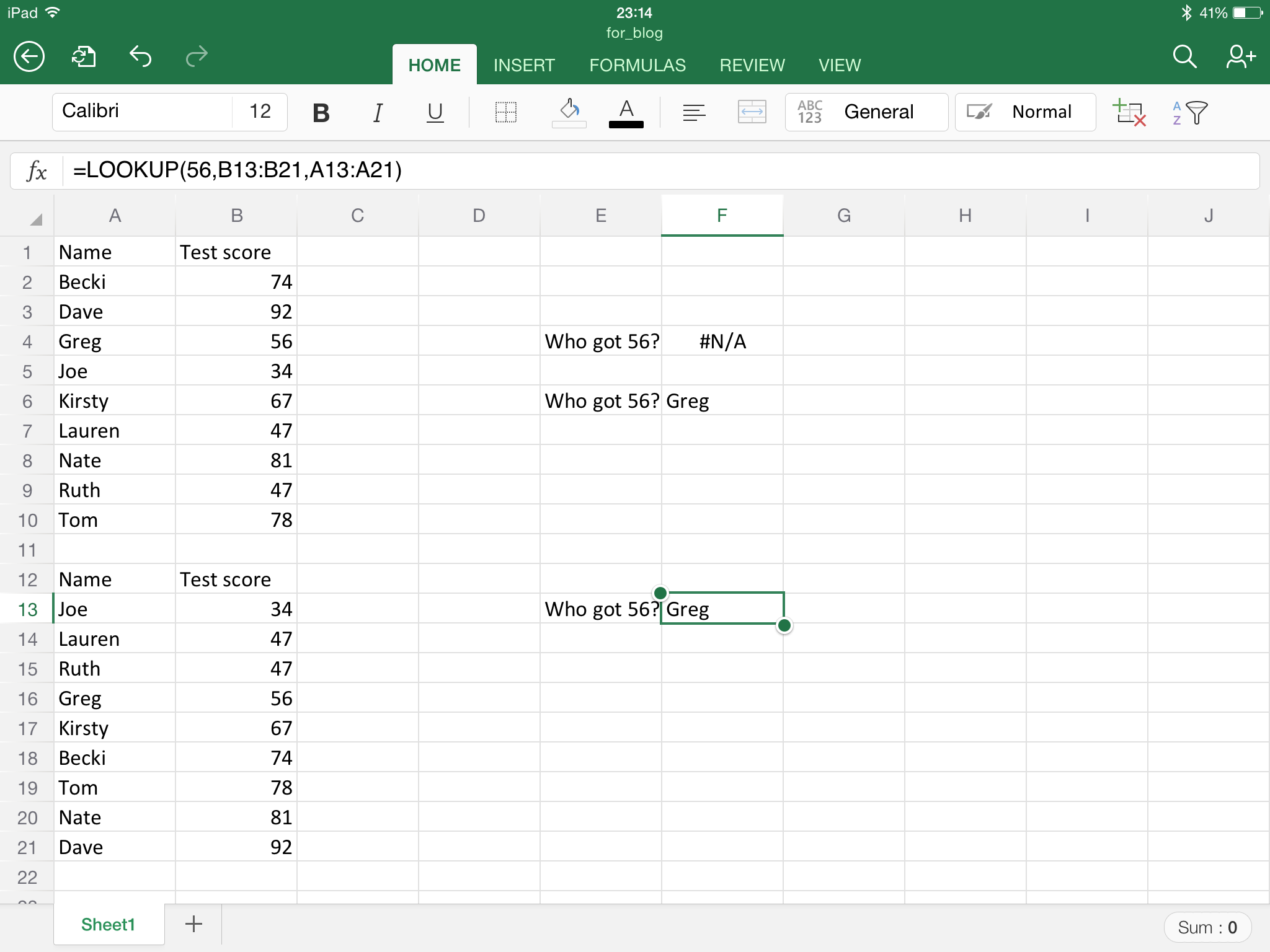In general I am not going to want to re-sort a table just to do a bit of analysis and then re-sort again at the end – that is just a massive waste of time.

Instead I prefer to combine the INDEX and MATCH functions that Excel provides. These functions do pretty much what you would expect, without any strange behaviour:

• MATCH returns the location of an item in an array. The first argument is the item we are searching for, the second the array in which we are searching and the third is an optional argument specifying if we are happy to accept a nearest match instead of an exact match.
• INDEX returns the value at a given location in an array. The first argument gives the array, the second tells which row of the array to return. There is an optional third argument that specifies a column number, for example if I wanted the entry in a table at the intersection of the 2nd row and 3r column.

Combining these two commands we can build a function that does not require data to be sorted into ascending order for the output to be trustworthy. This is shown in the following screen shot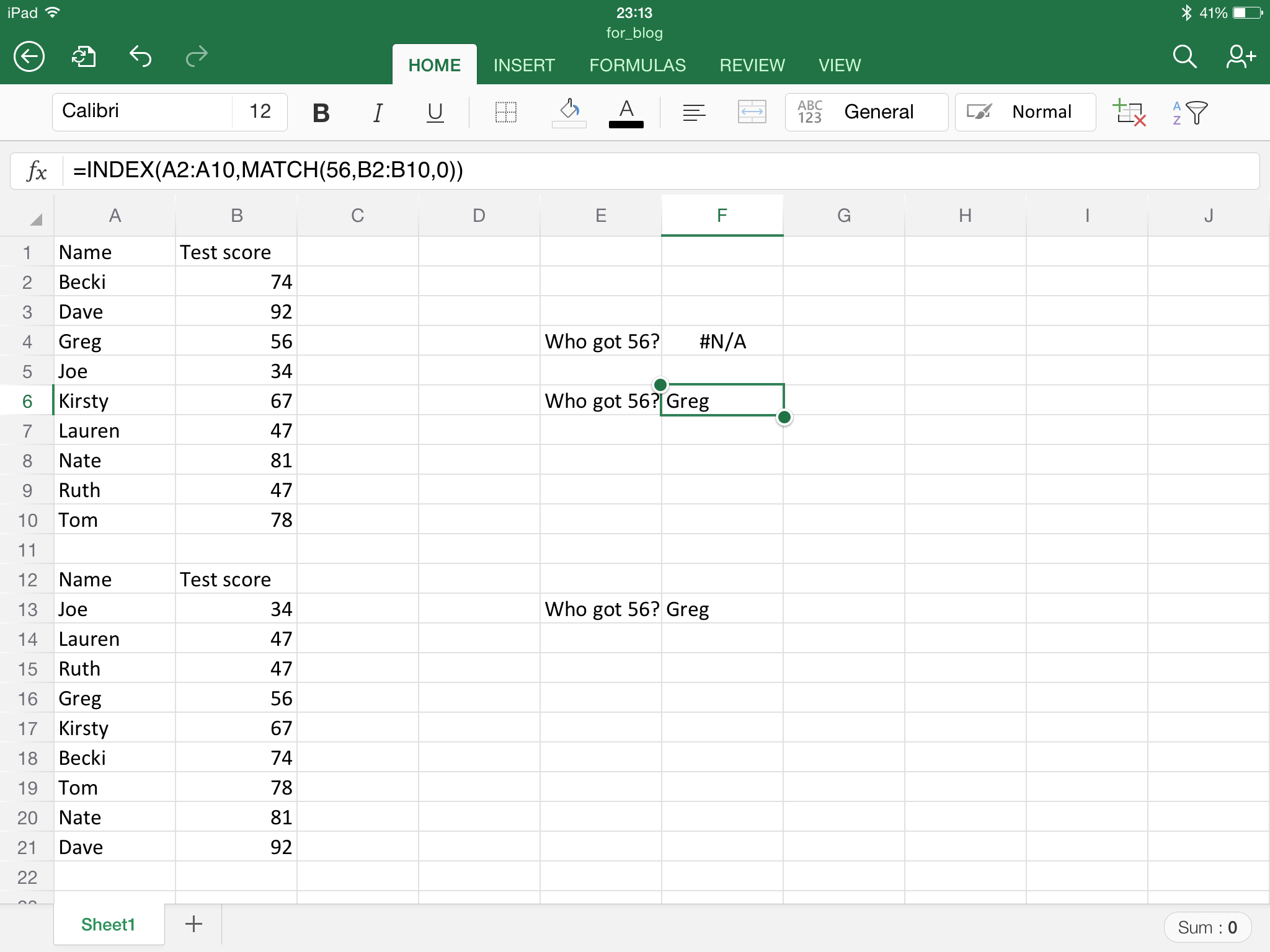I think I will now very rarely use the LOOKUP function, or VLOOKUP.

As an aside, the screen shots shown are from the iOS version of Excel, which actually has pretty good functionality. I’m not a fan of Microsoft products, being a Mac guy, but the iOS Microsoft Office apps are actually really good. I’m trying to shift to doing a much work as possible on my iPad now (not being able to always find a computer at school in free periods) and I’m sure I will blog about other apps that I find particularly good for the iPad.